# Data Structures in Python – Complete Guide

## What is Data Structure?

Data structure refers to the computational storage of data for efficient use. It stores the data in a way that can be easily modified and accessed. It collectively refers to the data values, relation between them, and the operations that can be carried on the data. The importance of data structure lies in its application for developing computer programs. As computer programs rely heavily on data, proper arrangement of the data for easy access is of foremost importance towards any program or software.

The four main functions of a data structure are

• To input information
• To process the information
• To maintain the information
• To retrieve the information

## Types of Data structures in Python

Several data structures are supported by Python for easy access and storage of data. Python data structures types can be classified as primitive and non-primitive data types. The former data types include Integers, Float, Strings, and Boolean, while the latter one is the array, list, tuples, dictionaries, sets, and Files. Therefore, data structures in python are both built-in data structures and user-defined data structures. The built-in data structure is referred to as the non-primitive data structure.

### Built-in Data structures

Python has several structures of data that act as containers for the storage of other data. These python data structures are List, Dictionaries, Tuple, and Sets.

### User-defined data structures

These data structures can be programmed as the same function as that of the built-in data structures in python. The user defined data structures are;Linked List, Stack, Queue, Tree, Graph, and Hashmap.

## List of in-build Data structuresand explanation

### 1. List

The data stored in a list are sequentially arranged and of different data types. For every data, an address is assigned and it is known as the index. Index value starts with a 0 and goes on till the last element. This is called a positive index. A negative index also exists if the elements are accessed reversely. This is called negative indexing.

List creation

The list is created as square brackets. Elements can then be added accordingly. It can be added within the square brackets to create a list. If no elements are added, an empty list will be created. Else the elements within the list will be created.

 Input my_list = [] #create empty list print(my_list) my_list = [1, 2, 3, ‘example’, 3.132] #creating list with data print(my_list) Output [] [1, 2, 3, ‘example’, 3.132]

Three functions are used for the addition of elements within a list. These functions are append(), extend(), and insert().

• All the elements are added as a single element using the append() function.
• For adding elements one by one in the list, the extend() function is used.
• For adding elements by their index value, the insert() function is used.
 Input my_list = [1, 2, 3] print(my_list) my_list.append([555, 12]) #add as a single element print(my_list) my_list.extend([234, ‘more_example’]) #add as different elements print(my_list) my_list.insert(1, ‘insert_example’) #add element i print(my_list) Output: [1, 2, 3] [1, 2, 3, [555, 12]] [1, 2, 3, [555, 12], 234, ‘more_example’] [1, ‘insert_example’, 2, 3, [555, 12], 234, ‘more_example’]

Deletion of elements within a list

A built-in keyword “del” in python is used to delete an element from the list. However, this function doesn’t return the deleted element.

• For returning a deleted element the pop() function is used. It uses the index value of the element to be deleted.
• The remove() function is used to delete an element by its value.

Output:

[1, 2, 3, ‘example’, 3.132, 30]

[1, 2, 3, 3.132, 30]

Popped Element: 2 List remaining: [1, 3, 3.132, 30]

[]

Assessing of elements in a list

• Assessing the element in a list is simple. Printing the list will directly display the elements.
• Specific elements can be assessed by passing the index value.

Output:

1

2

3

example

3.132

10

30

[1, 2, 3, ‘example’, 3.132, 10, 30]

Example

[1, 2]

[30, 10, 3.132, ‘example’, 3, 2, 1]

In addition to the above-mentioned operations, several other in-built functions are available in python for working with lists.

• len(): the function is used to return the length of the list.
• index(): this function allows the user to know the index value of a value passed.
• count() function is used to find the count of the value passed to it.
• sort() sorts the value in a list and modifies the list.
• sorted() sorts the value in a list and returns the list.

Output

6

3

2

[1, 2, 3, 10, 10, 30]

[30, 10, 10, 3, 2, 1]

### 2. Dictionary

Dictionary is a type of data structure where key-value pairs are stored rather than single elements. It can be explained with the example of a phone directory which has all the numbers of individuals along with their Phone numbers. The name and phone number here defines the constant values that are the “key” and the numbers and names of all the individuals as the values to that key. Assessing a key will give access to all the values stored within that key. This defined key-value structure on Python is known as a dictionary.

Creation of a dictionary

• The flower braces idle the dict() function can be used for creating a dictionary.
• The key-value pairs are to be added while creating a dictionary.

Modification in key-value pairs

Any modifications in the dictionary can be done only through the key. Therefore, the keys should be accessed first and then the modifications are to be carried out.

 Input my_dict = {‘First’: ‘Python’, ‘Second’: ‘Java’} print(my_dict) my_dict[‘Second’] = ‘C++’ #changing element print(my_dict) my_dict[‘Third’] = ‘Ruby’ #adding key-value pair print(my_dict) Output: {‘First’: ‘Python’, ‘Second’: ‘Java’} {‘First’: ‘Python’, ‘Second’: ‘C++’} {‘First’: ‘Python’, ‘Second’: ‘C++’, ‘Third’: ‘Ruby’}

Deletion of a dictionary
A clear () function is used to delete the whole dictionary. The dictionary can be assessed through the keys using the get() function or passing the key values.

 Input dict = {‘Month’: ‘January’, ‘Season’: ‘winter’} print(dict[‘First’])  print(dict.get(‘Second’) Output January Winter

Other functions associated with a dictionary are keys(), values(), and items().

### 3. Tuple

Similar to the list, Tuples are data storage lists, but the only difference is that the data stored in a tuple cannot be modified. If the data within a tuple is mutable, only then it’s possible to change the data.

• Tuples can be created through the tuple() function.

Input

new_tuple = (10, 20, 30, 40)

print(new_tuple)

Output

(10, 20, 30, 40)

• Elements in a tuple can be assessed in the same manner as assessing elements in a list.

Input

new_tuple2 = (10, 20, 30, ‘age’)

for x in new_tuple2:

print(x)

print(new_tuple2)

print(new_tuple2)

Output

10

20

30

Age

(10, 20, 30, ‘age’)

10

• ‘+’ operator is used to append another tuple

Input

tuple = (1, 2, 3)

tuple = tuple + (4, 5, 6

print(tuple)

Output

(1, 2, 3, 4, 5, 6)

4. Set

The set data structure is similar to the arithmetic sets. It is basically the collection of unique elements. If the data keeps on repeating, then sets consider adding that element only once.

• A set can be created just by passing the values to it within flower braces.

Input

set = {10, 20, 30, 40, 40, 40}

print(set)

Output

{10, 20, 30, 40}

• The add() function can be used to add elements to a set.
• To combine data from two sets, the union() function can be used.
• To identify the data which is present in both sets, intersection() function is used.
• The difference() function outputs only the data that is unique to the set, removing the common data.
• The symmetric_difference() function outputs the data unique to both sets.

### List of user-defined data structures and explanation

#### 1. Stacks

A stack is a linear structure that is either a Last in First out (LIFO) or a First in Last Out (FIFO) structure. Two main operations exist in the stack i.e. push and pop. Push means the appending of an element in the top of the list whereas pop means removing an element from the bottom of the stack. The process is well described in Figure 1.

Usefulness of stack

• Previous elements can be assessed through backward tracing.
• Matching of recursive elements.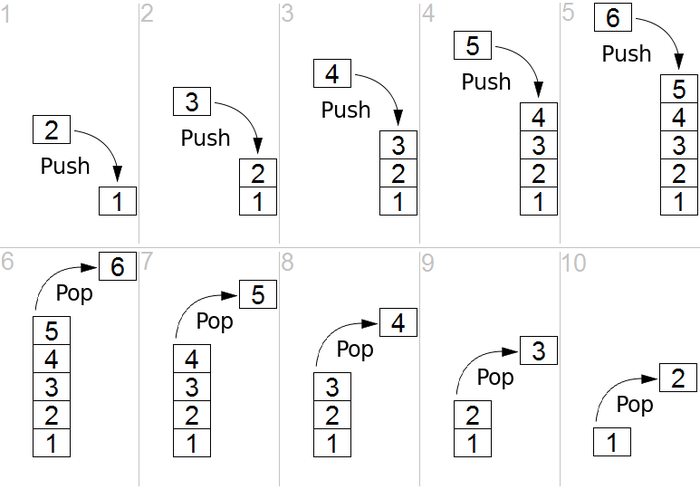Source

Figure 1: Graphical representation of Stack

Example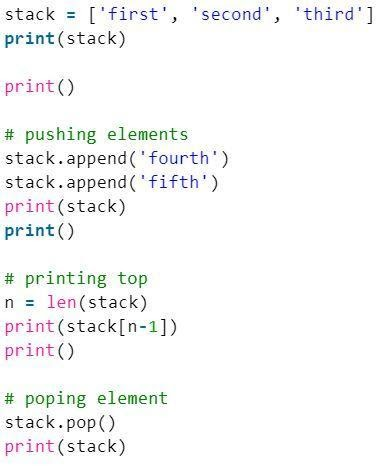Output

[‘first’, ‘second’, ‘third’]

[‘first’, ‘second’, ‘third’, ‘fourth’, ‘fifth’]

fifth

[‘first’, ‘second’, ‘third’, ‘fourth’]

#### 2. Queue

Similar to the stacks, a queue is a linear structure that allows the insertion of an element at one end and deletion from the other end. The two operations are known as enqueue and dequeue. The recently added element is removed first like the stacks. A graphical representation of the queue is shown in Figure 2. One of the main uses of a queue is for the processing of things as soon as they enter.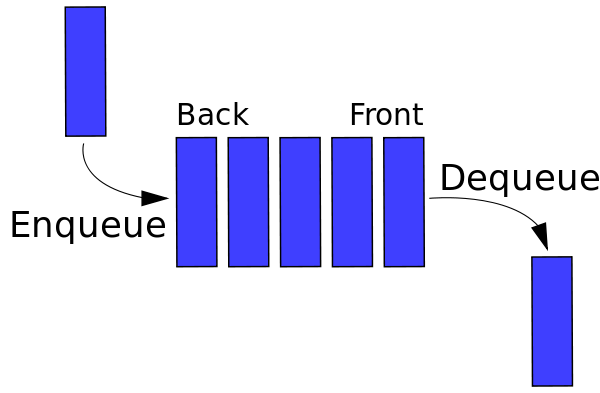Source

Figure 2: Graphical representation of Queues

Example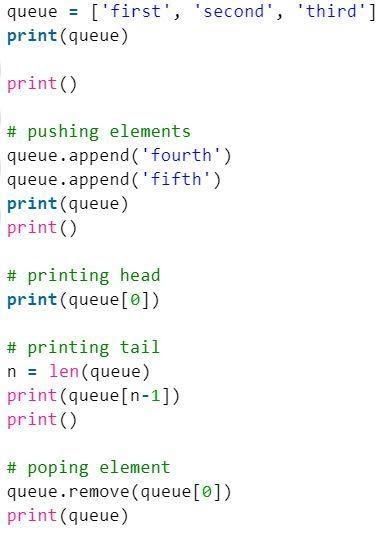Output

[‘first’, ‘second’, ‘third’]

[‘first’, ‘second’, ‘third’, ‘fourth’, ‘fifth’]

first

fifth

[‘second’, ‘third’, ‘fourth’, ‘fifth’]

#### 3. Tree

Trees are non-linear and hierarchical data structures consisting of nodes linked through edges. The python tree data structure has a root node, parent node, and child node. The root is the topmost element of a data structure. A binary tree is a structure in which elements have no more than two child nodes.

The usefulness of a tree

• Displays the structural relationships of the data elements.
• Traversal through each node efficiently
• The users can insert, search, retrieve and delete the data.
• Flexible data structures

Figure 3: Graphical representation of a tree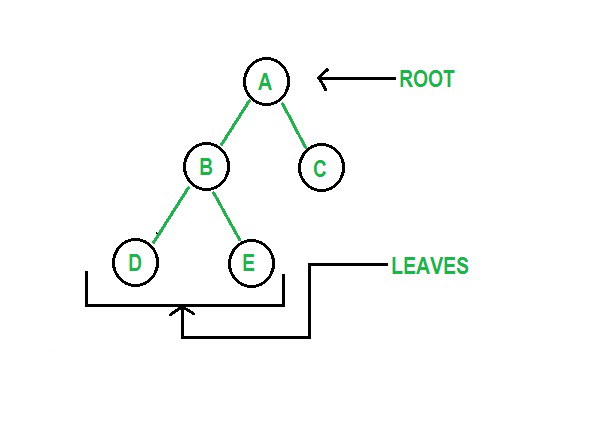Source

Example: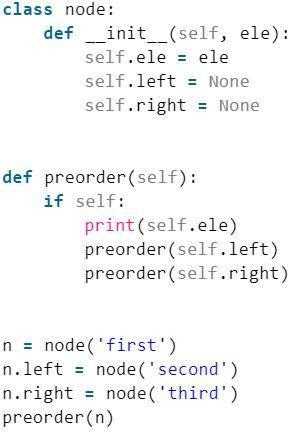Output

First

Second

Third

4. Graph

Another non-linear data structure in python is the graph that consists of nodes and edges. Graphically it displays a set of objects, with some objects connected through links. The vertices are interconnected objects while the links are termed as edges. Representation of a graph can be done through the dictionary data structure of python, where the key represents the vertices and the values represent the edges.

Basic operations that can be performed on graphs

• Display graph vertices and edges.
• Creation of a graph

The usefulness of a Graph

• The representation of a graph is easy to understand and follow.
• It is a great structure to represent linked relationships i.e. Facebook friends.

Figure 4: Graphical representation of a graph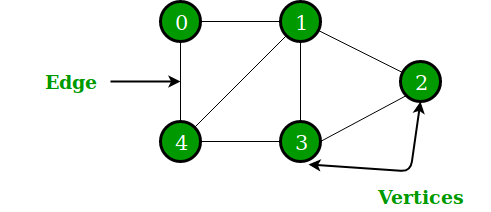Source

Example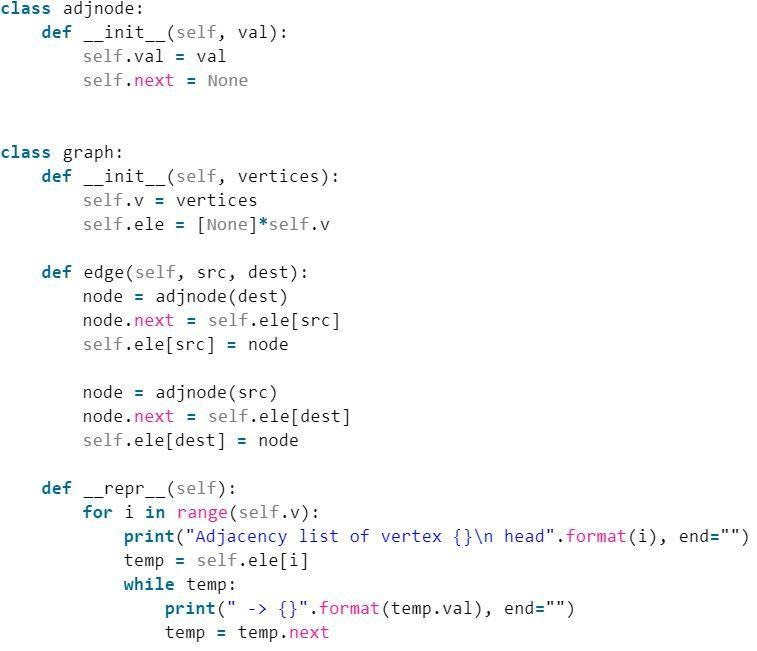g = graph(4)

g.edge(0, 2)

g.edge(1, 3)

g.edge(3, 2)

g.edge(0, 3)

g.__repr__()

Output

head -> 0 -> 2 -> 1

#### 5. Hashmap

Hashmaps are indexed python data structures useful for the storage of key-value pairs. Data stored in hashmaps are retrieved through the keys which are computed through the help of a hash function. These types of data structures are useful for the storage of student data, customer details, etc. Dictionaries in python are an example of hashmaps.

Example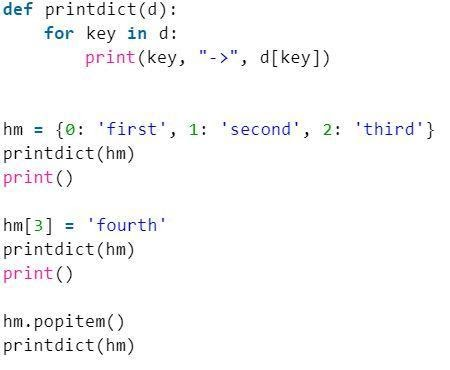Output

0 -> first

1 -> second

2 -> third

0 -> first

1 -> second

2 -> third

3 -> fourth

0 -> first

1 -> second

2 -> third

Usefulness

• It is the most flexible and reliable method of retrieving information than other data structures.

It is a type of linear data structure. Basically, it is a series of data elements joined together through links in python. Elements in a linked list are connected through pointers. The first node of this data structure is referred to as the header and the last node is referred to as the tail. Therefore, a linked list consists of nodes having values, and each node consists of a pointer linked to another node.

• Compared to an array which is fixed, a linked list is a dynamic form of data entry. Memory is saved as it allocates the memory of the nodes. While in an array, the size has to be predefined, leading to memory wastage.
• A Linked list can be stored anywhere in the memory. A linked list node can be updated and moved to a different location.

Figure 6: Graphical representation of a Linked List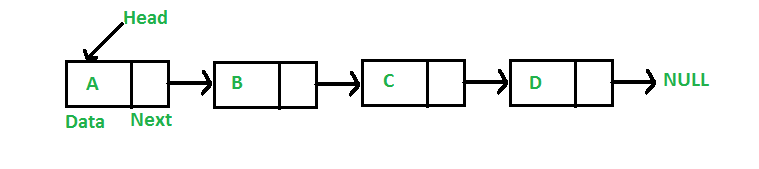Source

Example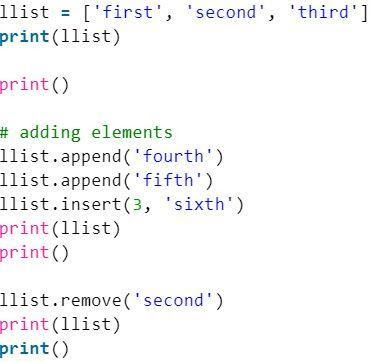Output:

[‘first’, ‘second’, ‘third’]

[‘first’, ‘second’, ‘third’, ‘sixth’, ‘fourth’, ‘fifth’]

[‘first’, ‘third’, ‘sixth’, ‘fourth’, ‘fifth’]

## Conclusion

The various types of data structures in python have been explored. Whether a novice or an expert the data structures and the algorithms can’t be ignored. While performing any form of operations on the data, the concepts of data structures play a vital role. The data structures help in storing the information in an organized manner, whereas the algorithms help in guiding throughout the data analysis. Therefore, both the python data structures and algorithms assist the computer scientist or any users to process their data.

If you are curious to learn about data structures, check out IIIT-B & upGrad’s Executive PG Program in Data Science which is created for working professionals and offers 10+ case studies & projects, practical hands-on workshops, mentorship with industry experts, 1-on-1 with industry mentors, 400+ hours of learning and job assistance with top firms.

### Which data structure is faster in Python?

In dictionaries, lookups are faster because Python uses hash tables to implement them. If we use Big O concepts to illustrate the distinction, dictionaries possess constant time complexity, O(1), whereas lists have linear time complexity, O(n).

In Python, dictionaries are the quickest way to lookup data with thousands of entries frequently. Dictionaries are highly optimised because they are the built-in mapping type in Python. In dictionaries and lists, however, there is a common space-time tradeoff. It indicates that while we can reduce the time required for our approach, we will need to use more memory space.

In lists, to get what you want, you must go through the full list. A dictionary, on the other hand, will return the value you're looking for without looking through all of the keys.

### Which is faster in Python list or array?

In general, Python lists are incredibly flexible and may hold entirely heterogeneous, random data, as well as being appended to quickly and in approximated constant time. They are the way to go if you need to downsize and extend your list quickly and painlessly. However, they take up a lot more space than arrays, partly because each item in the list necessitates the creation of a separate Python object.

On the other hand, the array.array type is essentially a thin wrapper around C arrays. It can only carry homogenous data (that is, data of the same type), hence memory is limited to sizeof(one object) * length bytes.

### What is the difference between NumPy array and list?

Numpy is Python's scientific computing core package. It uses a large multidimensional array object as well as utilities for manipulating them. A numpy array is a grid of identical-type values indexed by a tuple of non-negative integers.

Lists were included in the Python core library. A list is similar to an array in Python, but it can be resized and contain elements of various types. What is the real difference here? Performance is the answer. Numpy data structures are more efficient in terms of size, performance, and functionality.

## Prepare for a Career of the Future

UPGRAD AND IIIT-BANGALORE'S EXECUTIVE PG PROGRAM IN DATA SCIENCE
Apply Now

## 0 replies on “Data Structures in Python – Complete Guide”